# Transforming vectors with matrices

### Transforming vectors with matrices

In this lesson, we will learn how to transform column vectors with matrices. To transform a vector, we need to multiply the transformation matrix with that vector. This would result in a transformed vector, where the length or direction of the vector may be changed. We will take a look at some questions which involve transforming vectors, and then graph them to notice the changes between the normal vector and the transformed vector.

#### Lessons

Let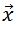be a column vector and $T$ be a transformation matrix. Then we can transform the column vectorby multiplying it with the transformation matrix $T$. In other words,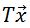is the transformed vector.
• Introduction
Transforming vectors with matrices overview

• 1.
Finding the Transformed Vectors
Apply the transformation matrix $T$ to the following column vectorto find the transformed vector:
a)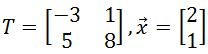b)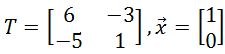c)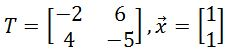d)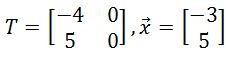e)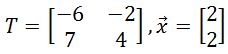• 2.
Graphing the Transformed Vector
Plot the column vector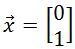on the graph. Then apply the transformation matrix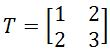to the column vector to find the transformed vector, and plot the transformed vector on the graph.

• 3.
Plot the column vector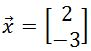on the graph. Then apply the transformation matrix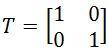to the column vector to find the transformed vector, and plot the transformed vector on the graph.

• 4.
Plot the column vector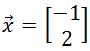on the graph. Then apply the transformation matrix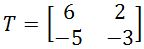to the column vector to find the transformed vector, and plot the transformed vector on the graph.